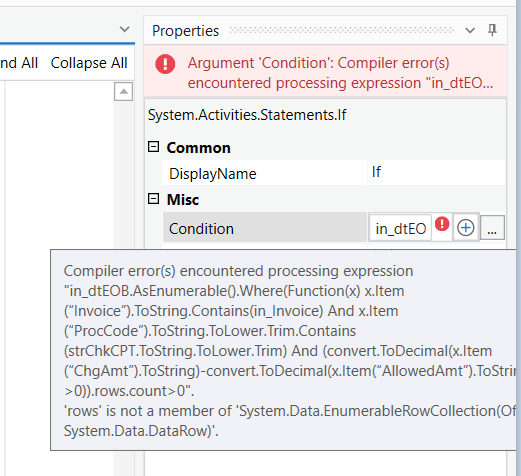# Condition error in if loop

Hi Forum Members,

I’m new to RPA and finding difficulty in one of my modules. Please do assist!

I have a Data table named in_dteob—it has columns (Slno, DOS, ProcCode, SubmittedCharge, AllowedAmount, Invoice)

when the invoice and proc code is matching with the application, I have to do the below subtraction

(Submiitedcharge-Allowedamount)

I’ve declared the variables for submittedcharge and allowed amount as string.

when I’m trying to do the subtraction I’m receiving the attached error. It would be helpful if someone assisted me.

Below is the Condition I used

in_dtEOB.AsEnumerable().Where(Function(x) x.Item(“Invoice”).ToString.Contains(in_Invoice) And x.Item(“ProcCode”).ToString.ToLower.Trim.Contains(strChkCPT.ToString.ToLower.Trim) And convert.ToDecimal(x.Item(“ChgAmt”).ToString)-convert.ToDecimal(x.Item(“AllowedAmt”).ToString))

Attached Error Snapshot

dtEOB.xlsx (8.4 KB)

Hi

Welcome to UiPath forum

As you have kept in IF activity the above expression must give a BOOLEAN Value as a output

And the mentioned one gives only the datatable

So mention like this

in_dtEOB.AsEnumerable().Where(Function(x) x.Item(“Invoice”).ToString.Contains(in_Invoice) And x.Item(“ProcCode”).ToString.ToLower.Trim.Contains(strChkCPT.ToString.ToLower.Trim) And (convert.ToDecimal(x.Item(“ChgAmt”).ToString)-convert.ToDecimal(x.Item(“AllowedAmt”).ToString)>0)).CopyToDatatable().Rows.Count > 0

Which means if the expression gives us any rows more than zero count then it will go to THEN part

Cheers @jaichithra.kanniyappan

I think the query will not work,

In your query, you are subtracting the two data after converting into decimals, and this will not produce a Boolean, may I know what you are doing with the subtraction.

If you are subtracting the values and comparing means it will be a Boolean and valid input for the query,

Like this example,

(convert.ToDecimal(x.Item(“ChgAmt”).ToString)-convert.ToDecimal(x.Item(“AllowedAmt”).ToString) =0)

thanks

@Palaniyappan I’m not going to copy the subtracted value to datatable. Once i got value, it should go to the then part where im creating a new variable and assigning it as true

In my case, the two data (submitted and allowed amount will come as decimals. so i need to convert them to decimals and do the subtraction.

If subtraction returns value, then i need to assign it as true by creating a variable.

any workaround pls!

in_dtEOB.AsEnumerable().Where(Function(x) x.Item(“Invoice”).ToString.Contains(in_Invoice) And x.Item(“ProcCode”).ToString.ToLower.Trim.Contains(strChkCPT.ToString.ToLower.Trim) And (convert.ToDecimal(x.Item(“ChgAmt”).ToString)-convert.ToDecimal(x.Item(“AllowedAmt”).ToString)>0)).rows.count>0

Thanks

@prasath_S now im getting attached newerror

@jaichithra.kanniyappan updated one

in_dtEOB.AsEnumerable().Where(Function(x) x.Item(“Invoice”).ToString.Contains(in_Invoice) And x.Item(“ProcCode”).ToString.ToLower.Trim.Contains(strChkCPT.ToString.ToLower.Trim) And (convert.ToDecimal(x.Item(“ChgAmt”).ToString)-convert.ToDecimal(x.Item(“AllowedAmt”).ToString)<>0)).count>0

Thanks

1 Like

Thank you @prasath_S , your solution worked for me !

1 Like

@jaichithra.kanniyappan Glad your issue is resolved,there seems to be a problem marking the solution, please mark my answer as solution once that issue is resolved,so it may be useful to others in the future.

Thanks

@prasath_S I’m new to this forum, can you tell where should I mark your post as solution.

1 Like

There seems to be issue in marking the solution at the moment once its resolved, it will let you know thanks…

For the reference this is where we have to check the solution button.

Thanks

1 Like

Thank you @prasath_S1 Like

I have mentioned the same which gives us count and if the count is greater than 0 it will give boolean as True which will take us to Then

Cheers @jaichithra.kanniyappan

2 Likes

Hi @Palaniyappan yes but the other thing missed is as I posted earlier The subtraction of two value is not producing a Boolean value that’s also the other error we have to consider I think.

thanks